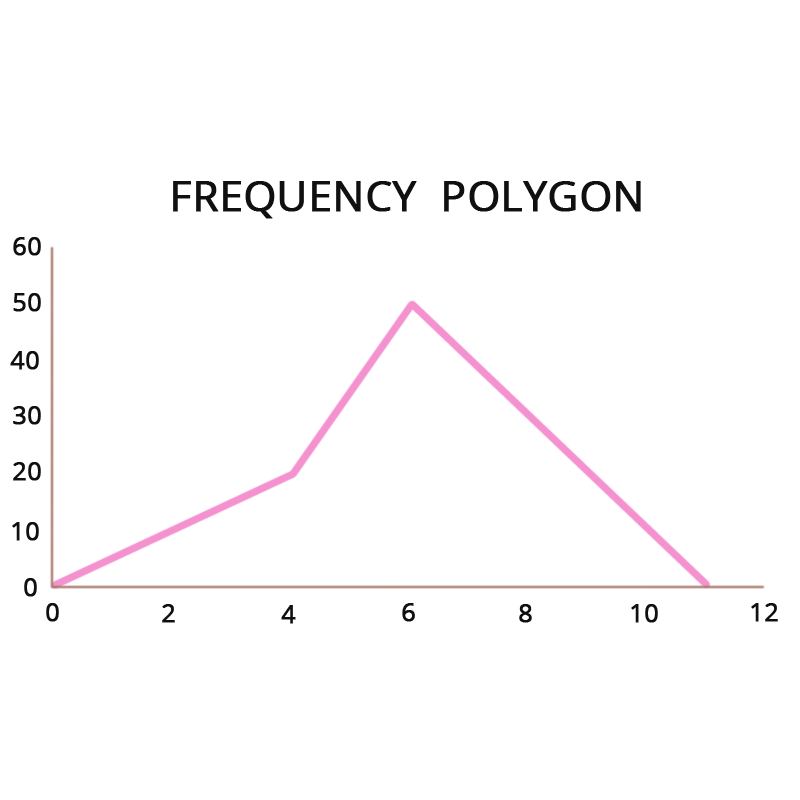• Call Now

1800-102-2727•

# Frequency Polygon

A frequency polygon is similar to a histogram and is used to compare data sets or depict a cumulative frequency distribution. It represents quantitative data with a line graph.

Statistics is concerned with the gathering of facts and information for a specific goal. In cricket, the statistics of the game are calculated by tabulating each run for each ball. Graphs, tables, pie charts, histograms, bar graphs, polygons, and other visual representations of statistical data are used.

Frequency polygons are a visually appealing way to display quantitative data and frequency distributions.

## What exactly is a frequency polygon?

The frequency polygon histogram resembles a column graph but without any gaps between columns. The frequency polygon is a type of line graph that is commonly used in statistics. These graphs can be drawn alone or in combination. For the creation of these graphs, the information contained in a frequency distribution table is used.

Let's look at how to draw a frequency polygon.

## How to Draw a Frequency Polygon

To draw frequency polygons, first, draw a histogram and then proceed as follows:

Step 1: Pick a class interval and write the values on the horizontal axis.

Step 2: On the horizontal axis, mark the midpoint of each period.

Step 3: On the vertical axes, write the frequency of the class.

Step 4: Mark a point in the middle of each class interval at the height that corresponds to the frequency of the class interval.

Step 5: Using the line segment, connect these places.

Step 6: The resultant representation is a frequency polygon.

Let's look at an example to help us understand this:The primary goal of statistics is to gather and arrange information in a sensible way. One of the fundamentals of data organizing is presenting data in a recognized format that can be easily comprehended. Tables may be used to arrange data, while pictures can be used to depict the data.

Bar charts, pie charts, histograms, and frequency polygons are examples of pictorial data display. The advantage here is that information in a graphic format is easier to comprehend at a glance.

## How can you tell the difference between a frequency polygon and a frequency curve?

The main difference between a frequency polygon and a frequency curve is that a frequency polygon is drawn by connecting points with a straight line, whereas a frequency curve is drawn by connecting points following a curve.Talk to our expert
Resend OTP Timer =
By submitting up, I agree to receive all the Whatsapp communication on my registered number and Aakash terms and conditions and privacy policy